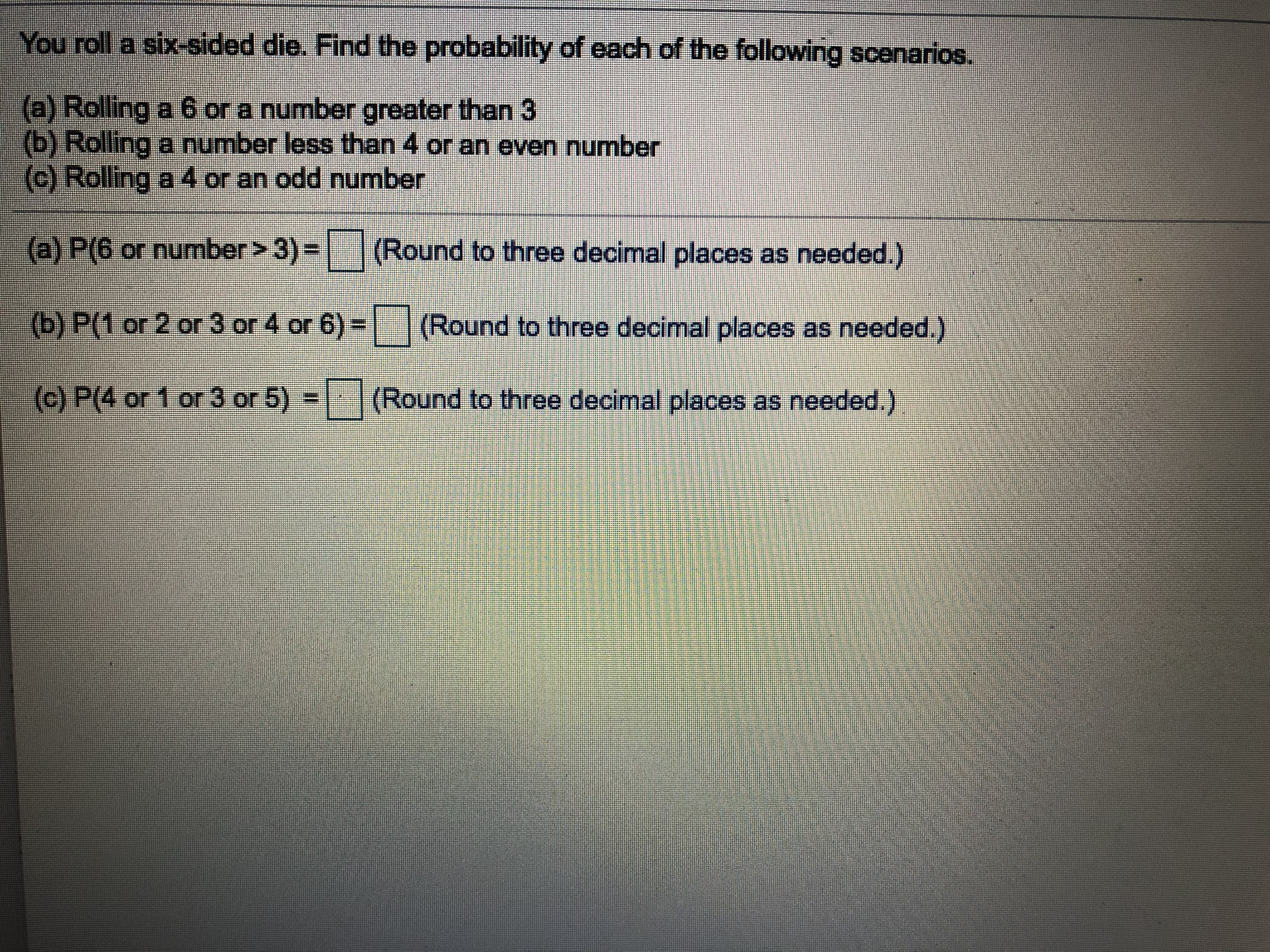# You roll a six-sided die. Find the probability of each of the following scenarios.(a) Rolling a 6 or a number greater than 3(b) Rolling a number less than 4 or an even number(c) Rolling a 4 or an odd number(a) P(6 or number> 3) =(Round to three decimal places as needed.)(b) P(1 or 2 or 3 or 4 or 6) =(Round to three decimal places as needed.)(c) P(4 or 1 or 3 or 5) =(Round to three decimal places as needed.)

Question
37 viewshelp_outlineImage TranscriptioncloseYou roll a six-sided die. Find the probability of each of the following scenarios. (a) Rolling a 6 or a number greater than 3 (b) Rolling a number less than 4 or an even number (c) Rolling a 4 or an odd number (a) P(6 or number> 3) = (Round to three decimal places as needed.) (b) P(1 or 2 or 3 or 4 or 6) = (Round to three decimal places as needed.) (c) P(4 or 1 or 3 or 5) = (Round to three decimal places as needed.) fullscreen
check_circle

Step 1

From the given question,

Sample space = {1, 2, 3, 4, 5, 6}

Consider,

A be the event that shows Rolling a six or number greater than 3

B be the event that shows number les...

### Want to see the full answer?

See Solution

#### Want to see this answer and more?

Solutions are written by subject experts who are available 24/7. Questions are typically answered within 1 hour.*

See Solution
*Response times may vary by subject and question.
Tagged in

### Other